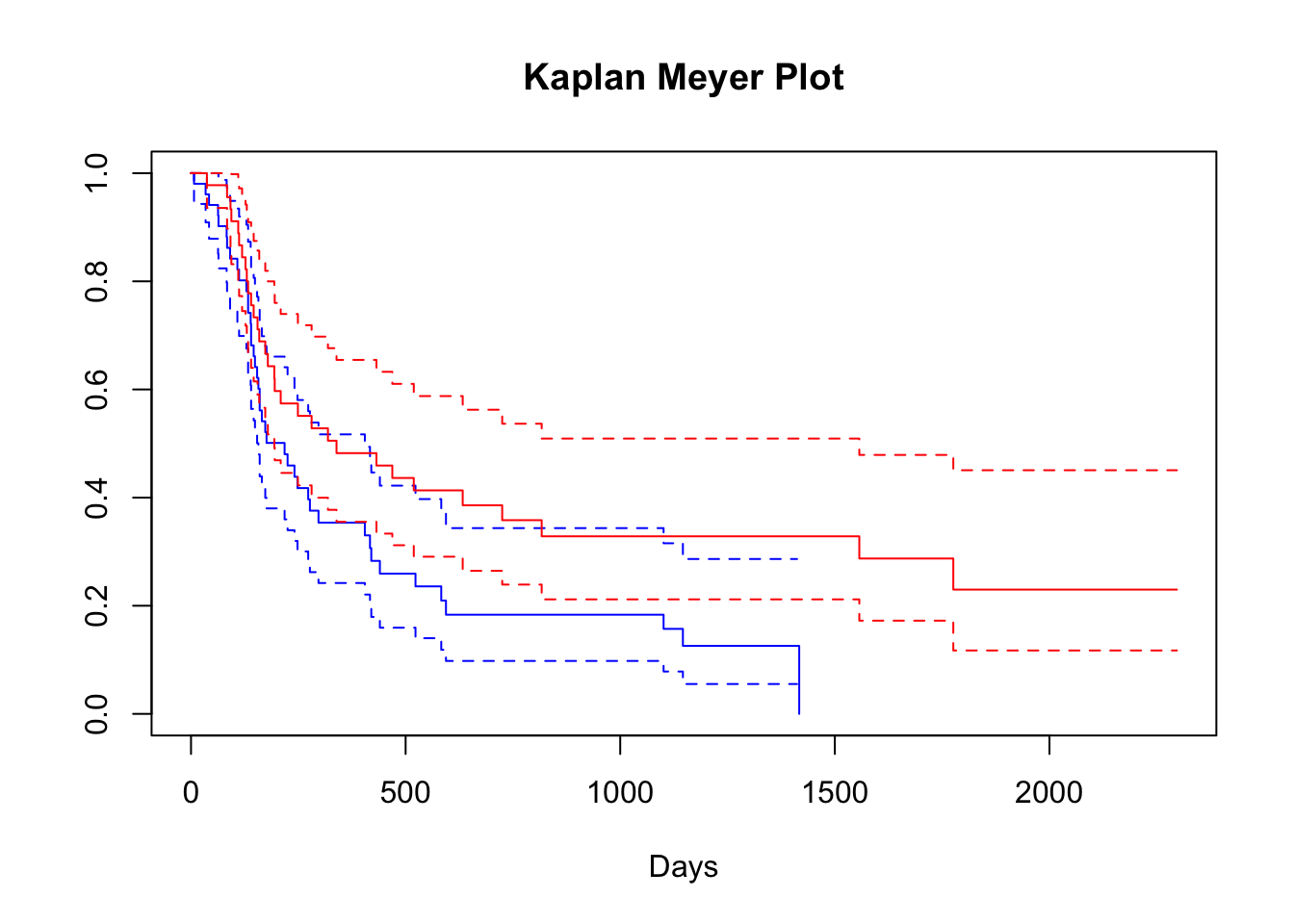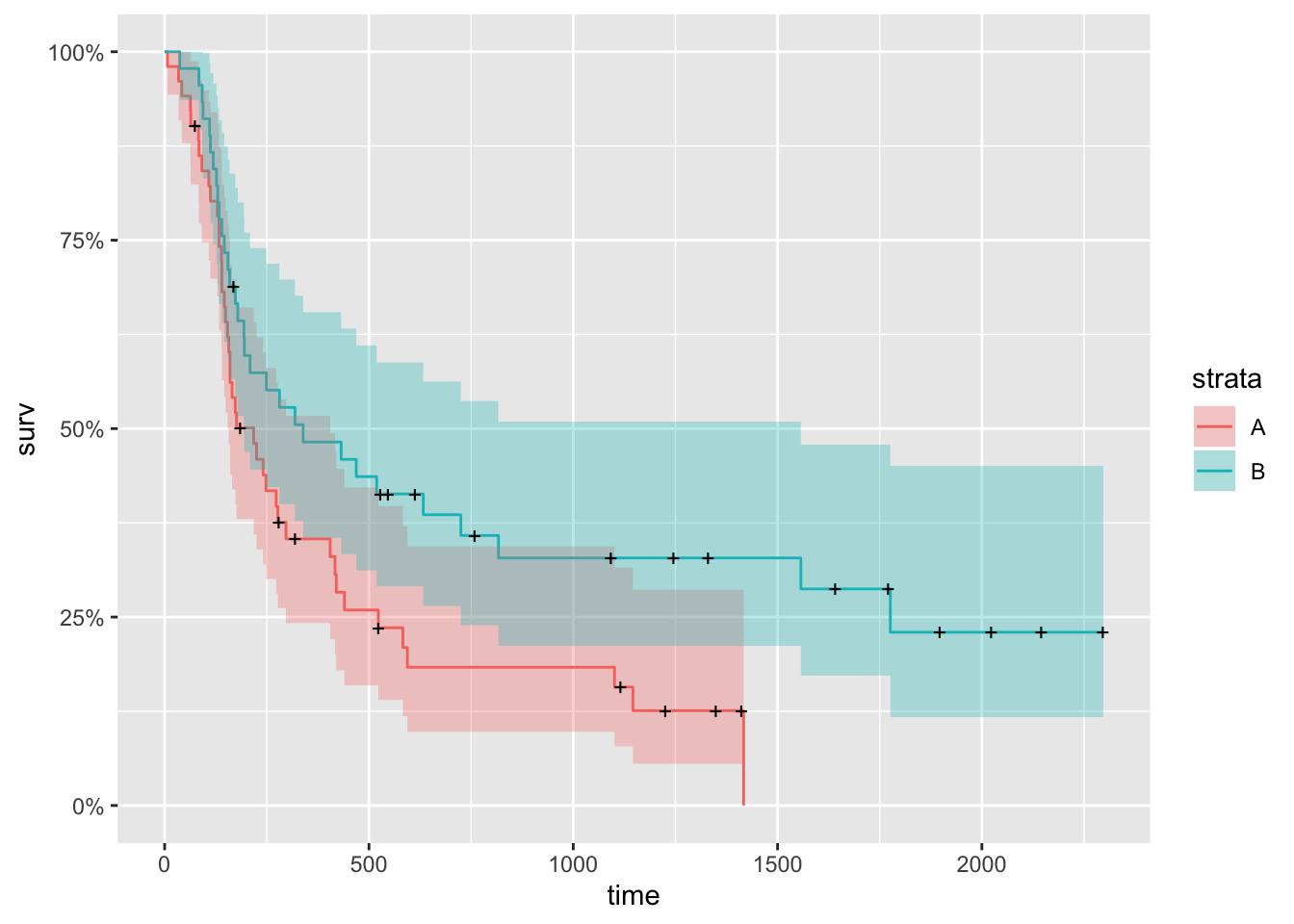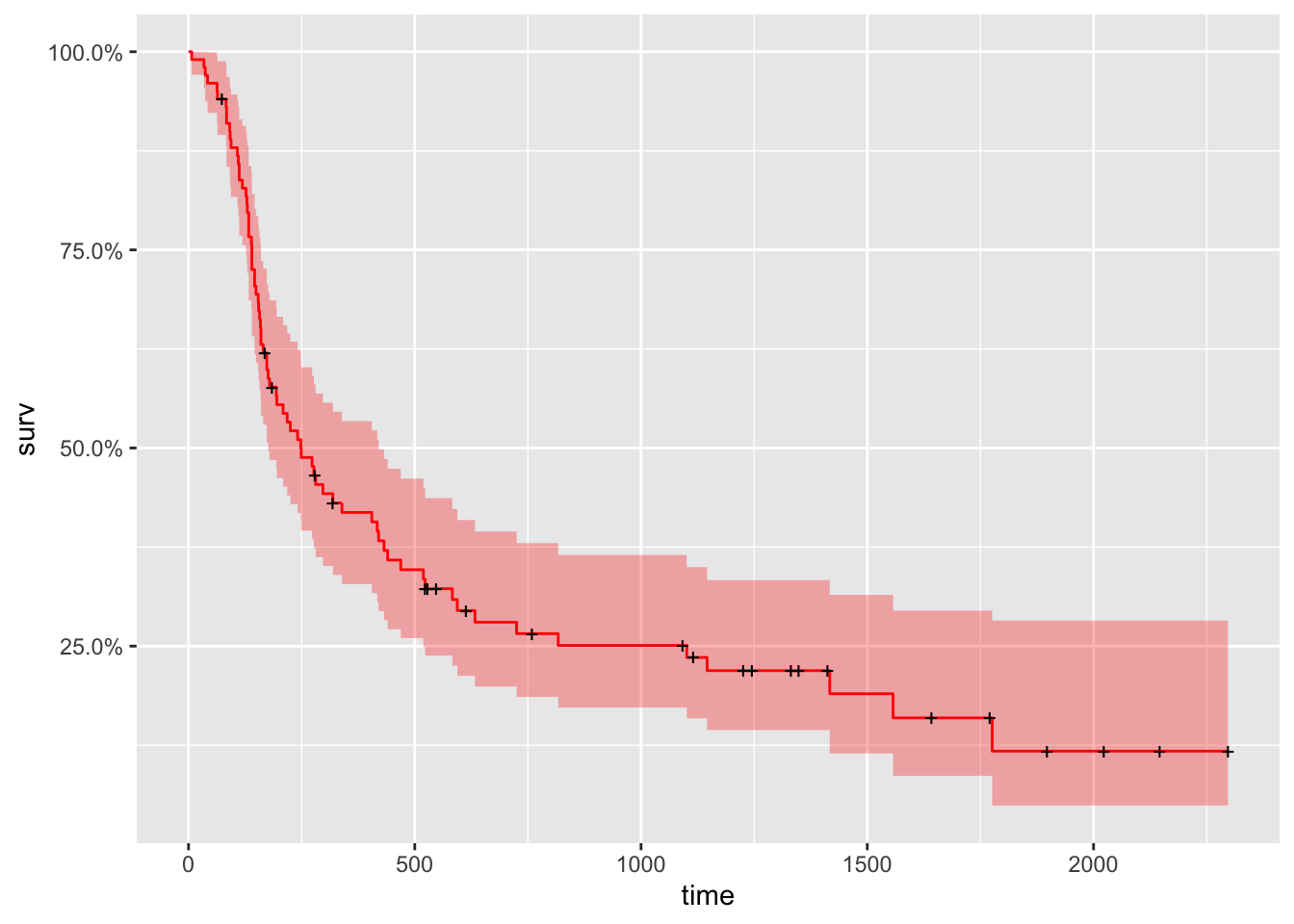# NCOG study

A randomized clinical trial conducted by the Northern California Oncology Group (NCOG) compared two treatments for head and neck cancer: chemotherapy (Arm A of the trial, $$n = 51$$ patients) and chemotherapy plus radiation (Arm B, $$n = 45$$ patients). The data records the survival time in number of days past treatment for each patient. The variable $$d = 0$$ represents that the patient is still alive on their final day of observation.

ncog <- read.table("https://web.stanford.edu/~hastie/CASI_files/DATA/ncog.txt", header = T)
ncog
• arm: treatment arms, A or B
• t: the survival time in number of days
• d: death (1) / censoring (0)

## 1. Kaplan-Meier survival curve

library(survival)
## Warning: package 'survival' was built under R version 3.6.2
km <- with(ncog, Surv(t, d))

km_fit <- survfit(Surv(t, d) ~ arm, data=ncog)
#summary(km_fit)

plot(km_fit, xlab="Days", main = 'Kaplan Meyer Plot', col = c("blue", "red"), conf.int = T)This shows a pointwise $$0.95$$ CI for the Kaplan Meier curve of each arm. We can have a more polished figure using ggplot2.

library(ggfortify)
## Warning: package 'ggfortify' was built under R version 3.6.2
## Loading required package: ggplot2
library(ggplot2)
autoplot(km_fit)The plus signs represent the censored cases at a given time point.

## 2. The log-rank test: comparison between two arms

surv_diff <- survdiff(Surv(t, d) ~ arm, data = ncog)
surv_diff
## Call:
## survdiff(formula = Surv(t, d) ~ arm, data = ncog)
##
##        N Observed Expected (O-E)^2/E (O-E)^2/V
## arm=A 51       42     32.5      2.77      5.24
## arm=B 45       31     40.5      2.22      5.24
##
##  Chisq= 5.2  on 1 degrees of freedom, p= 0.02

The function returns a list of components, including:

• N: the number of subjects in each group.
• obs: the observed number death in each group
• exp: the expected number of death in each group under the null.

Here the p-value is $$0.02$$, indicating a rejection of the null that the two survival functions are the same in the two arms.

## 3. The proportional hazards regression model

cox <- coxph(Surv(t, d) ~ day + month + year + arm, data = ncog)
summary(cox)
## Call:
## coxph(formula = Surv(t, d) ~ day + month + year + arm, data = ncog)
##
##   n= 96, number of events= 73
##
##           coef exp(coef) se(coef)      z Pr(>|z|)
## day    0.01219   1.01227  0.01471  0.829   0.4074
## month  0.01094   1.01100  0.03401  0.322   0.7477
## year   0.03593   1.03659  0.07496  0.479   0.6317
## armB  -0.53677   0.58464  0.24601 -2.182   0.0291 *
## ---
## Signif. codes:  0 '***' 0.001 '**' 0.01 '*' 0.05 '.' 0.1 ' ' 1
##
##       exp(coef) exp(-coef) lower .95 upper .95
## day      1.0123     0.9879    0.9835    1.0419
## month    1.0110     0.9891    0.9458    1.0807
## year     1.0366     0.9647    0.8950    1.2006
## armB     0.5846     1.7105    0.3610    0.9469
##
## Concordance= 0.577  (se = 0.039 )
## Likelihood ratio test= 6.15  on 4 df,   p=0.2
## Wald test            = 6.02  on 4 df,   p=0.2
## Score (logrank) test = 6.15  on 4 df,   p=0.2
cox_fit <- survfit(cox)
autoplot(cox_fit, surv.colour = "red")This is the predicted survival curve for a single “pseudo” subject with covariate values equal to the means of the data set (often not really make sense in practice).